# Math in Focus Grade 3 Chapter 15 Practice 3 Answer Key Measuring Capacity

This handy Math in Focus Grade 3 Workbook Answer Key Chapter 15 Practice 3 Measuring Capacity provides detailed solutions for the textbook questions.

## Math in Focus Grade 3 Chapter 15 Practice 3 Answer Key Measuring Capacity

Find the capacity of each container.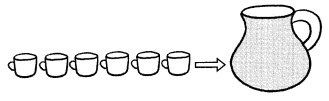Question 1.
The pitcher can hold _________ cups of water.
The pitcher can hold 6 cups of water.
Explanation:
In the above image we can observe 6 cups and a pitcher. So, the pitcher can hold 6 cups of water.

Question 2.
The pitcher has a capacity of __________ cups.
The pitcher has a capacity of 6 cups.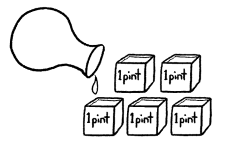Question 3.
The vase can hold __________ pints of water.
The vase can hold 5 pints of water.
Explanation:
In the above image we can observe 5 pints and a vase. The vase can hold 5 pints of water.

Question 4.
The vase has a capacity of ________ pints.
The vase has a capacity of 5 pints.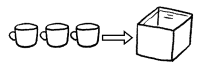Question 5.
The carton can hold __________ cups of milk.
The carton can hold 3 cups of milk.
Explanation:
In the above image we can observe 3 cups and a carton. The carton can hold 3 cups of milk.

Question 6.
The carton has a capacity of __________ cups.
The carton has a capacity of 3 cups.

Find the capacity of each container.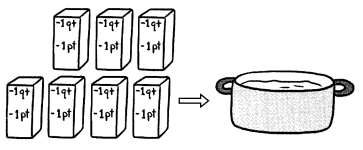Question 7.
The pot can hold __________ quarts of soup.
The pot can hold 7 quarts of soup.
Explanation:
In the above we can observe 7 quarts of soup and a pot. We know that 1 quarts is equal to 2 pints. The pot can hold 7 quarts of soup.

Question 8.
The pot has a capacity of __________ quarts.
The pot has a capacity of 7 quarts.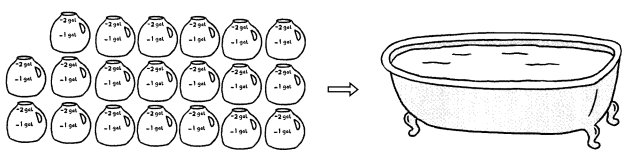Question 9.
The bathtub can hold __________ gallons of water.
The bathtub can hold 40 gallons of water.
Explanation:
In the above image we can observe 20 pots containing 2 gallons of water each. So, the bathtub can hold 40(20 x 2) gallons of water.

Question 10.
The bathtub has a capacity of __________ gallons.
The bathtub has a capacity of 40 gallons.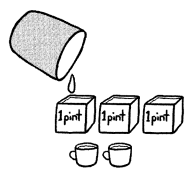Question 11.
This container can hold __________ cups of juice.
This container can hold 8 cups of juice.
Explanation:
In the above image we can observe 3 pints and 2 cups of juice and a container.
We know that 1 pint is equal to 2 cups.
2 + 2 + 2 + 2 = 8
The above container can hold 8 cups of juice.

Question 12.
This container has a capacity of __________ cups.
This container has a capacity of 8 cups.

Question 13.
Match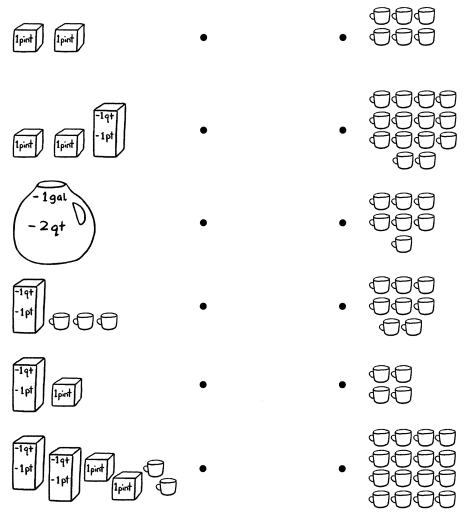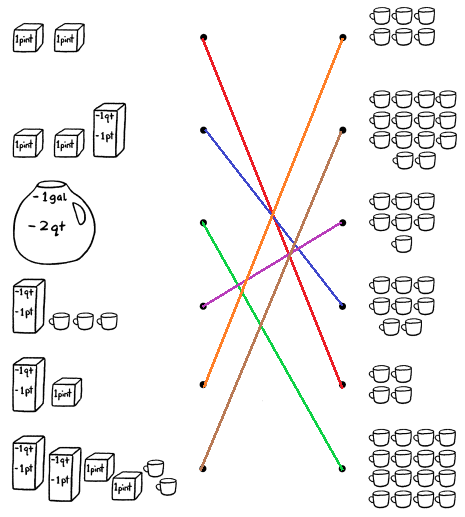Explanation:
We know that 1 pint is equal to 2 cups.
1 quarts is equal to 2 pints, which is equal to 4 cups.
1 gallon is equal to 4 quarts, which is equal to 16 cups.
In the above image, we can observe

• 2 pints in the first diagram. So, we need to map it to  2 + 2 = 4 cups.
• 2 pints and 1 quarts in the second diagram. So, we need to map it to 2 + 2 + 4 = 8 cups.
• 1 gallon in the third diagram. So, we need to map it to 4 x 2 x 2 = 16 cups.
• 1 quarts and 3 cups in fourth diagram. So, we need to map it to 4 + 3 = 7 cups.
• 1 quarts and 1 pint in fifth diagram. So, we need to map it to 4 + 2 = 6 cups.
• 2 quarts and 2 pints and 2 cups. So, we need to map it to 4 + 4 + 2 + 2 + 2 = 14 cups.

Cross out (✗) the one that does not have the same capacity.

Question 14.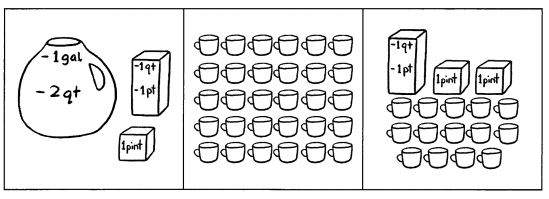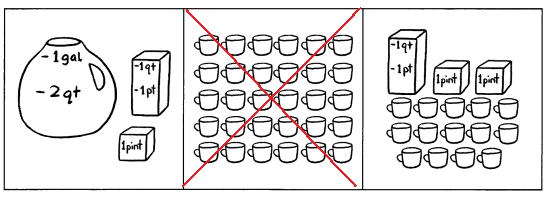Explanation:
We know that 1 pint is equal to 2 cups.
1 quarts is equal to 2 pints, which is equal to 4 cups.
1 gallon is equal to 4 quarts, which is equal to 16 cups.
In the above image, we can observe

• 1 gallon, 1 quarts and 1 pint in first part.
16 cups + 4 cups + 2 cups = 22 cups
• 30 cups in second part.
• 1 quarts, 2 pints and 14 cups in third part.
4 cups + 2 cups + 2cups + 14 cups = 22 cups
• Second part doesn’t have same capacity. So, cross out the second part.
• Question 15.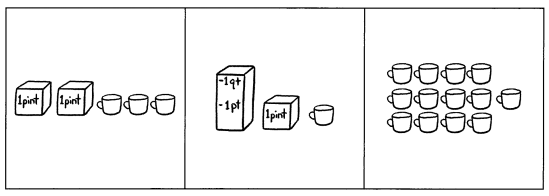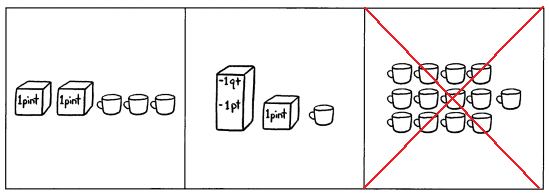Explanation:
We know that 1 pint is equal to 2 cups.
1 quarts is equal to 2 pints, which is equal to 4 cups.
1 gallon is equal to 4 quarts, which is equal to 16 cups.
In the above image, we can observe
• 2 pints and 3 cups in first part.
2 cups + 2 cups + 3 cups = 7 cups
• 1 quarts, 1pint and 1 cup in second part.
4 cups + 2 cups + 1 cup = 7 cups
• 13 cups in third part.
• Third part doesn’t have same capacity. So, cross out the third part.

Question 16.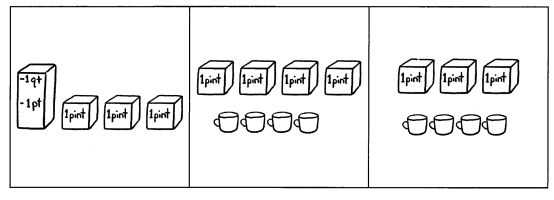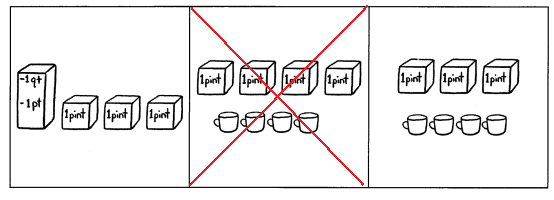Explanation:
We know that 1 pint is equal to 2 cups.
1 quarts is equal to 2 pints, which is equal to 4 cups.
1 gallon is equal to 4 quarts, which is equal to 16 cups.
In the above image, we can observe

• 1 quarts, and 3 pint in first part.
4 cups + 2 cups + 2 cups + 2cups = 10 cups
• 4 pints and 4 cups in second part.
8 cups + 4 cups = 12 cups
• 3 pints and 4 cups in third part.
6 cups + 4 cups = 10 cups
• Second part doesn’t have same capacity. So, cross out the second part.